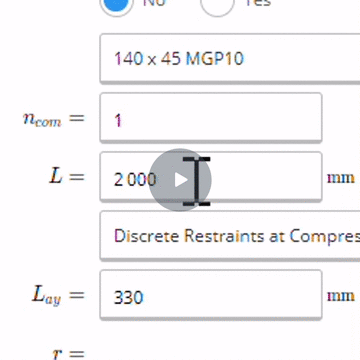# How to Use Formulas and Convert Units in Inputs

Just like in Excel, ClearCalcs lets you perform quick calculations inside any input cell. Unlike Excel however, ClearCalcs also lets you type units and converts them on the fly! All you need to do is enter the mathematical operation you want, and you can include any units you'd like!

# Use a Formula

1. Simply start typing a formula into any input field
2. Type in your preferred formula (for example $= 5 \cdot 1500$)
3. ClearCalcs will automatically perform the calculation, including

See it in action# Convert Units

Units may be used in any input field. You can even perform calculations with units, such as $$EI = 200 GPa \cdot 50.0e6 mm^3$$ . ClearCalcs recognises hundreds of different types of units, and if you're using the metric system, all the standard SI prefixes (u, m, c, k, M, G, etc.). Some examples of common units are listed below, but many others are also available - if you want to enter a length in "rods" or a volume in "teaspoons", you can do that too! First, a few general notes: